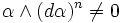# Contact manifold

## Definition

A contact manifold is the following data:

• A differential manifold$M$ of dimension$(2n + 1)$
• A hyperplane field (viz a smooth association of a tangent hyperplane at every point) that occurs as the kernel of a smooth 1-form$\alpha$ on$M$ satisfying:$\alpha \wedge (d\alpha)^n \ne 0$

Note that$\alpha$ is uniquely determined upto a scalar multiple.

### Terminology

• A smooth 1-form$\alpha$ described as above is termed a contact form
• A hyperplane field described as above is termed a contact field
• The overall datum is termed a contact structure